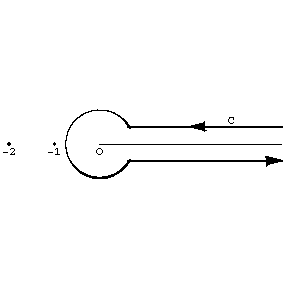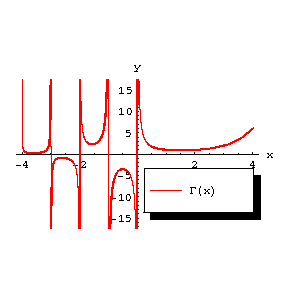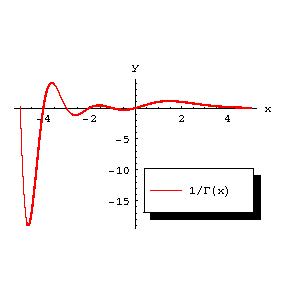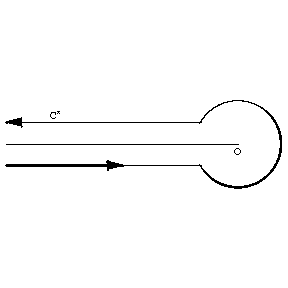# Talk:Gamma-function

$\Gamma$-function

$\newcommand{\abs}{\left|#1\right|} \newcommand{\Re}{\mathop{\mathrm{Re}}} \newcommand{\Im}{\mathop{\mathrm{Im}}}$

A transcendental function $\Gamma(z)$ that extends the values of the factorial $z!$ to any complex number $z$. It was introduced in 1729 by L. Euler in a letter to Ch. Goldbach, using the infinite product $$\Gamma(z) = \lim_{n\rightarrow\infty}\frac{n!n^z}{z(z+1)\ldots(z+n)} = \lim_{n\rightarrow\infty}\frac{n^z}{z(1+z/2)\ldots(1+z/n)},$$ which was used by L. Euler to obtain the integral representation (Euler integral of the second kind, cf. Euler integrals) $$\Gamma(z) = \int_0^\infty x^{z-1}e^{-x} \rd x,$$ which is valid for $\Re z > 0$. The multi-valuedness of the function $x^{z-1}$ is eliminated by the formula $x^{z-1}=e^{(z-1)\ln x}$ with a real $\ln x$. The symbol $\Gamma(z)$ and the name gamma-function were proposed in 1814 by A.M. Legendre.

If $\Re z < 0$ and $-k-1 < \Re z < -k$, $k=0,1,\ldots$, the gamma-function may be represented by the Cauchy–Saalschütz integral: $$\Gamma(z) = \int_0^\infty x^{z-1} \left( e^{-x} - \sum_{m=0}^k (-1)^m \frac{x^m}{m!} \right) \rd x.$$ In the entire plane punctured at the points $z=0,-1,\ldots$, the gamma-function satisfies a Hankel integral representation: $$\Gamma(z) = \frac{1}{e^{2\pi iz} - 1} \int_C s^{z-1}e^{-s} \rd s,$$ where $s^{z-1} = e^{(z-1)\ln s}$ and $\ln s$ is the branch of the logarithm for which $0 < \arg\ln s < 2\pi$; the contour $C$ is represented in Fig. a. [FIXME] It is seen from the Hankel representation that $\Gamma(z)$ is a meromorphic function. At the points $z_n = -n$, $n=0,1,\ldots$ it has simple poles with residues $(-1)^n/n!$.Figure: g043310a

## Fundamental relations and properties of the gamma-function.

1) Euler's functional equation: $$z\Gamma(z) = \Gamma(z+1),$$ or $$\Gamma(z) = \frac{1}{z\ldots(z+n)}\Gamma(z+n+1);$$ $\Gamma(1)=1$, $\Gamma(n+1) = n!$ if $n$ is an integer; it is assumed that $0! = \Gamma(1) = 1$.

2) Euler's completion formula: $$\Gamma(z)\Gamma(1-z) = \frac{\pi}{\sin \pi z}.$$ In particular, $\Gamma(1/2)=\sqrt{\pi}$; $$\Gamma\left(n+\frac{1}{2}\right) = \frac{1.3\ldots(2n-1)}{2^n}\sqrt{\pi}$$ if $n>0$ is an integer; $$\abs{\Gamma\left(\frac{1}{2} + iy\right)}^2 = \frac{\pi}{\cosh y\pi},$$ where $y$ is real.

3) Gauss' multiplication formula: $$\prod_{k=0}^{m-1} \Gamma\left( z + \frac{k}{m} \right) = (2\pi)^{(m-1)/2}m^{(1/2)-mz}\Gamma(mz), \quad m = 2,3,\ldots$$ If $m=2$, this is the Legendre duplication formula.

4) If $\Re z \geq \delta > 0$ or $\abs{\Im z} \geq \delta > 0$, then $\ln\Gamma(z)$ can be asymptotically expanded into the Stirling series: $$\ln\Gamma(z) = \left(z-\frac{1}{2}\right)\ln z - z + \frac{1}{2}\ln 2\pi + \sum_{n=1}^m \frac{B_{2n}}{2n(2n-1)z^{2n-1}} + O\bigl(z^{-2m-1}\bigr), \quad m = 1,2,\ldots,$$ where $B_{2n}$ are the Bernoulli numbers. It implies the equality $$\Gamma(z) = \sqrt{2\pi} z^{z-1/2} z^{-z} \left( 1 + \frac{1}{12}z^{-1} + \frac{1}{288}z^{-2} - \frac{139}{51840}z^{-3} - \frac{571}{2488320}z^{-4} + O\bigl(z^{-5}\bigr) \right).$$ In particular, $$\Gamma(1+x) = \sqrt{2\pi} x^{x+1/2} e^{-x + \theta/12x}, \quad 0 < \theta < 1.$$ More accurate is Sonin's formula [So]: $$\Gamma(1+x) = \sqrt{2\pi} x^{x+1/2} e^{-x + 1/12(x+\theta)}, \quad 0 < \theta < 1/2.$$

5) In the real domain, $\Gamma(x) > 0$ for $x > 0$ and it assumes the sign $(-1)^{k+1}$ on the segments $-k-1 < x < -k$, $k = 0,1,\ldots$ (Fig. b).Figure: g043310b

The graph of the function .

For all real $x$ the inequality $$\Gamma\Gamma^{\prime\prime} > \bigl(\Gamma^\prime\bigr)^2 \geq 0$$ is valid, i.e. all branches of both $\abs{\Gamma(x)}$ and $\ln\abs{\Gamma(x)}$ are convex functions. The property of logarithmic convexity defines the gamma-function among all solutions of the functional equation $$\Gamma(1+x) = x\Gamma(x)$$ up to a constant factor (see also the Bohr–Mollerup theorem).

For positive values of $x$ the gamma-function has a unique minimum at $x=1.4616321\ldots$ equal to $0.885603\ldots$. The local minima of the function $\abs{\Gamma(x)}$ form a sequence tending to zero as $x\rightarrow -\infty$.Figure: g043310c

The graph of the function .

6) In the complex domain, if $\Re z > 0$, the gamma-function rapidly decreases as $\abs{\Im z} \rightarrow \infty$, $$\lim_{\abs{\Im z} \rightarrow \infty} \abs{\Gamma(z)}\abs{\Im z}^{(1/2)-\Re z}e^{\pi\abs{\Im z}/2} = \sqrt{2\pi}.$$

7) The function $1/\Gamma(z)$ (Fig. c) is an entire function of order one and of maximal type; asymptotically, as $r \rightarrow \infty$, $$\ln M(r) \sim r \ln r,$$ where $$M(r) = \max_{\abs{z} = r} \frac{1}{\abs{\Gamma(z)}}.$$ It can be represented by the infinite Weierstrass product: $$\frac{1}{\Gamma(z)} = z e^{\gamma z} \prod_{n=1}^\infty \left(\left( 1 + \frac{z}{n} \right) e^{-z/n} \right),$$ which converges absolutely and uniformly on any compact set in the complex plane ($\gamma$ is the Euler constant). A Hankel integral representation is valid: $$\frac{1}{\Gamma(z)} = \frac{1}{2\pi i} \int_{C'} e^s s^{-z} \rd s,$$ where the contour $C'$ is shown in Fig. d.Figure: g043310d



G.F. Voronoi [Vo] obtained integral representations for powers of the gamma-function.

In applications, the so-called poly gamma-functions — $k$th derivatives of $\ln\Gamma(z)$ — are of importance. The function (Gauss' $\psi$-function) $$\psi(z) = \frac{\mathrm{d}}{\mathrm{d}z}\ln\Gamma(z) = \frac{\Gamma'(z)}{\Gamma(z)} = -\gamma + \sum_{n=0}^\infty \frac{z-1}{(n+1)(z+n)} = -\gamma + \int_0^1 \frac{1 - (1-t)^{z-1}}{t} \rd t$$ is meromorphic, has simple poles at the points $z=0,-1,\ldots$ and satisfies the functional equation $$\psi(z+1) - \psi(z) = \frac{1}{z}.$$ The representation of $\psi(z)$ for $\abs{z}<1$ yields the formula $$\ln\Gamma(1+z) = -\gamma z + \sum_{k=2}^\infty \frac{(-1)^k S_k}{k} z^k,$$ where $$S_k = \sum_{n=1}^\infty n^{-k}.$$ This formula may be used to compute $\Gamma(z)$ in a neighbourhood of the point $z=1$.

For other poly gamma-functions see [BaEr]. The incomplete gamma-function is defined by the equation $$I(x,y) = \int_0^y e^{-t}t^{x-1} \rd t.$$ The functions $\Gamma(z)$ and $\psi(z)$ are transcendental functions which do not satisfy any linear differential equation with rational coefficients (Hölder's theorem).

The exceptional importance of the gamma-function in mathematical analysis is due to the fact that it can be used to express a large number of definite integrals, infinite products and sums of series (see, for example, Beta-function). In addition, it is widely used in the theory of special functions (the hypergeometric function, of which the gamma-function is a limit case, cylinder functions, etc.), in analytic number theory, etc.